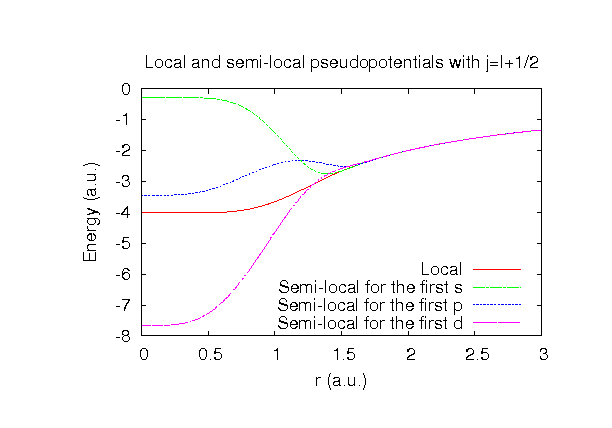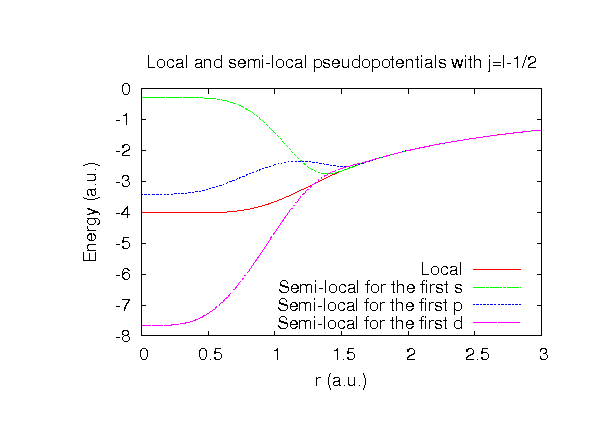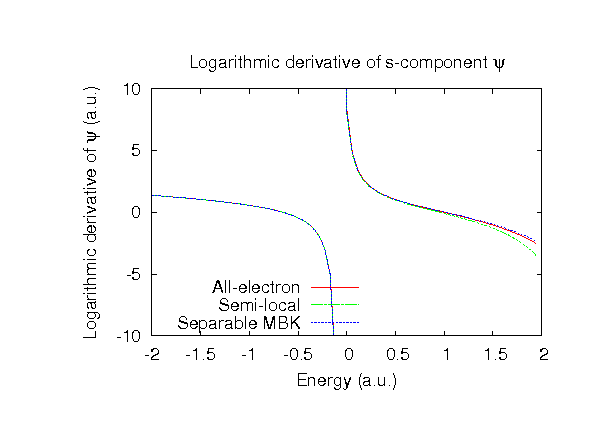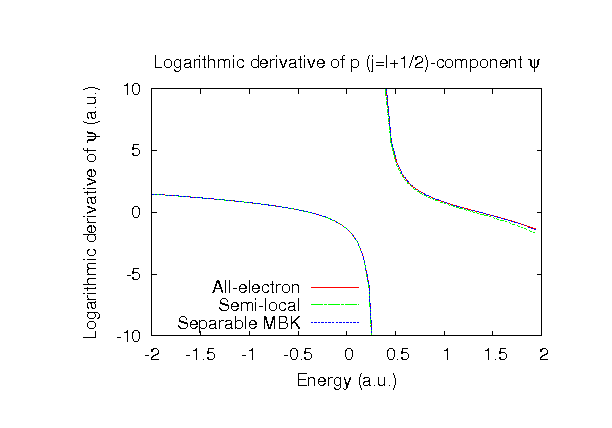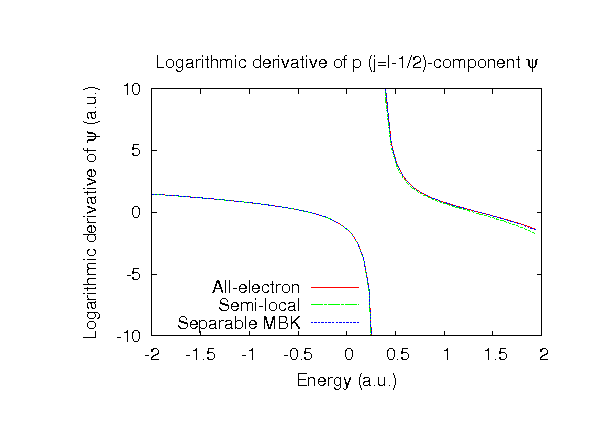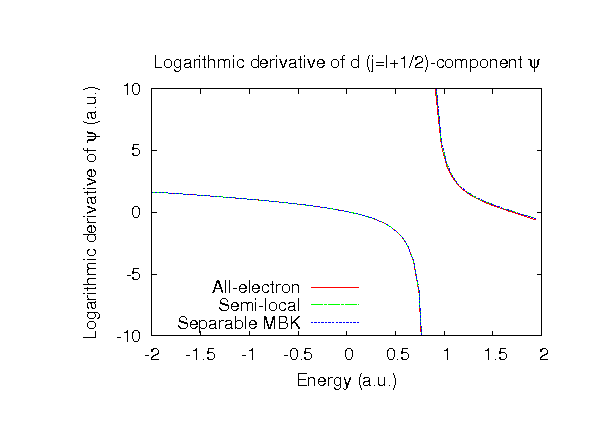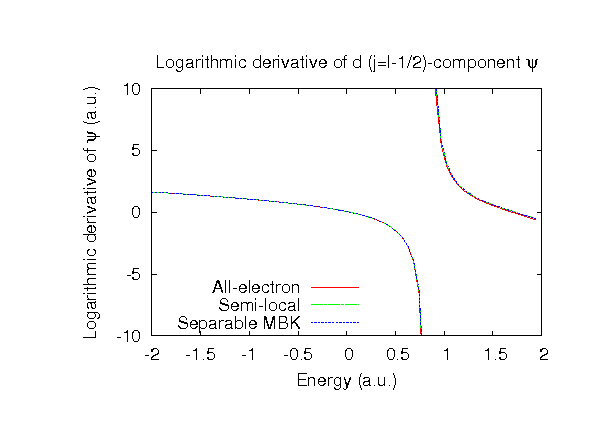### Fully relativistic pseudopotentials

Fully relativistic pseudopotentials generated by the MBK (PRB 47, 6728 (1993)) scheme within LDA (CA13) and GGA (PBE13) which contain a partial core correction and fully relativistic effects including spin-orbit coupling.

### Pseudo-atomic orbitals

The number below the symbol means a cutoff radius (a.u.) of the confinement potential. These file includes fifteen radial parts for each angular momentum quantum number l (=0,1,2,3). The basis functions were generated by variationally optimizing the corresponding primitive basis functions in the single atom and the dimer molecule. The input files used for the orbital optimization can be found at Si_opt.dat and Si2_opt.dat . Since Si_CA13.vps and Si_PBE13.vps include the 3s and 3p states (4 electrons) as the valence states, the minimal basis set is Si*.*-s1p1. Our recommendation for the choice of cutoff radius of basis functions is that Si7.0.pao is enough for bulks, but Si8.0.pao or Si9.0.pao is preferable for molecular systems.

### Benchmark calculations by the PBE13 pseudopotential with the various basis functions

(1) Calculation of the total energy as a function of lattice constant in the diamond structure, where the total energy is plotted relative to the minimum energy for each case. a0 and B0 are the equilibrium lattice constant and bulk modulus obtained by fitting to the Murnaghan equation of state. The difference between Si7.0-s2p2d1 and Si7.0-s3p3d2f1 in the total energy at the minimum point is 0.025 eV/atom. An input file used for the OpenMX calculations can be found at Sidia-EvsV.dat . For comparison the result by the Wien2k code is also shown, where the calculation was performed by default setting in the Ver. 10.1 of Wien2k.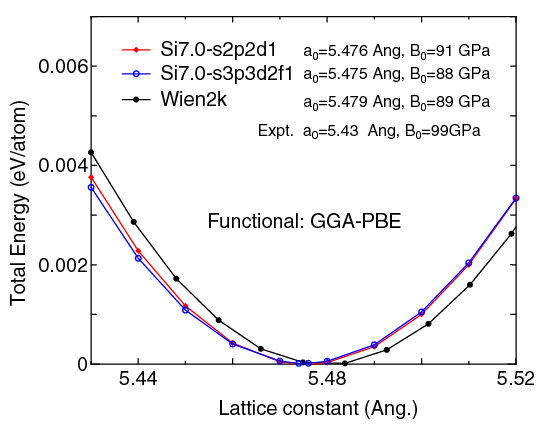(2) Calculations of the band dispersion in the diamond structure, where the non-spin polarized collinear calculation with the lattice constant of 5.43 Ang. was performed using Si_PBE13.vps and Si7.0-s2p2d1 or Si7.0-s3p3d2f1, and the Fermi level is taken to be the top of valence band. The input file used for the OpenMX calculations can be found at Sidia-Band.dat . For comparison the result by the Wien2k code is also shown, where the calculation was performed by default setting in the Ver. 10.1 of Wien2k.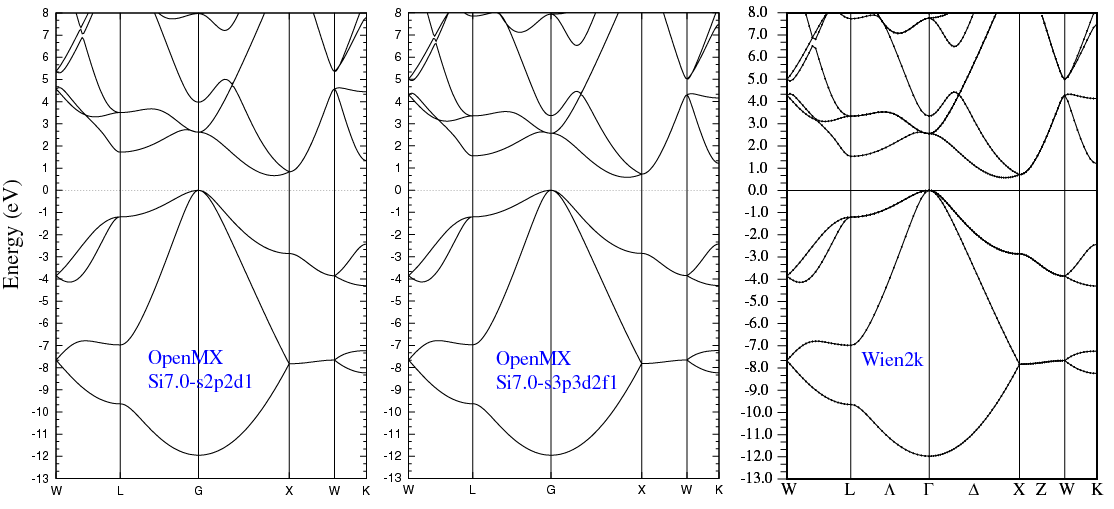(3) Calculations of a SiO molecule, where Si_PBE13.vps, O_PBE13.vps, Si9.0-s2p2d1, Si9.0-s3p3d2f1, O7.0-s2p2d1, and O7.0-s3p3d2f1 were used. The input files used for the OpenMX calculations can be found at SiO.dat , Si_SiO_1.dat , O_SiO_1.dat , Si_SiO_2.dat , O_SiO_2.dat , Si_SiO_cp1.dat , O_SiO_cp1.dat , Si_SiO_cp2.dat , and O_SiO_cp2.dat .

 Equilibrium bond length (Ang.) Dipole moment (Debye) Atomization energy (kcal/mol) Atomization energy (couterpoise corrected) (kcal/mol) Si9.0-s2p2d1, O7.0-s2p2d1 1.539 3.22 194.76 194.32 Si9.0-s3p3d2f1, O7.0-s3p3d2f1 1.540 3.06 196.55 196.45 Other calc. 1.54 a 191.4 a Expt. 1.51b 3.0983c 189 d

a A.C. Reber et al., ACS NANO 2, 1729 (2008).
b G. Herzberg, Molecular Spectra and Molecular Structure, vol. I, Van Nostrand, Princeton, 1950.
c J.W. Raymonda, J.S. Muenter, W.A. Klemperer, J. Chem. Phys. 52 (1970) 3458.
d D.E. Gray, Americal Institute of Physics Handbook (McGraw-Hill, New York, 1972).

### Supplementary information for the GGA (PBE13) pseudopotential